# Perpendicular Distance Of A Point From A Plane

The shortest distance of a point from a plane is said to be along the line perpendicular to the plane or in other words, is the perpendicular distance of the point from the plane. Thus, if we take the normal vector say ň to the given plane, a line parallel to this vector that meets the point P gives the shortest distance of that point from the plane. If we denote the point of intersection (say R) of the line touching P, and the plane upon which it falls normally, then the point R is the point on the plane that is the closest to the point P. Here, the distance between the point P and R gives the distance of the point P to the plane. In the upcoming discussion, we shall study about the calculation of the shortest distance of a point from a plane using the Vector method and the Cartesian Method.

### Vector form

Let us consider a point A whose position vector is given by ȃ and a plane P, given by the equation,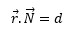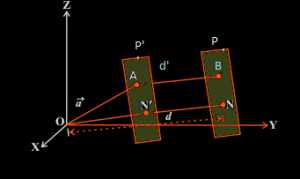Here, N is normal to the plane P under consideration. Now, let O be the origin of the coordinate system being followed and P’ another plane parallel to the first plane, which is taken such that it passes through the point A. Here, N’ is normal to the second plane. The equation of the second plane P’ is given by,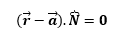Or,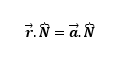We see that, the ON gives the distance of the plane P from the origin and ON’ gives the distance of the plane P’ from the origin. Thus, the distance between the two planes is given as,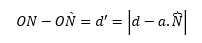This also given the perpendicular distance of the point A on plane P’ from the plane P.

Thus we conclude that, for a plane given by the equation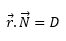, and a point A, with a position vector given by , the perpendicular distance of the point from the given plane is given by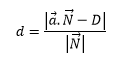In order to calculate the length of the plane from the origin, we substitute the position vector by 0, and thus it comes out to be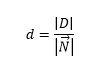### Cartesian form

Let us consider a plane given by the Cartesian equation,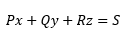And a point whose position vector is ȃ and the Cartesian coordinate is,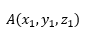We can write the position vector as: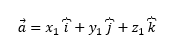In order to find the distance of the point A from the plane using the formula given in the vector form, in the previous section, we find the normal vector to the plane, which is given as,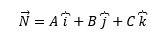Using the formula, the perpendicular distance of the point A from the given plane is given as,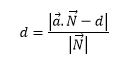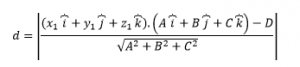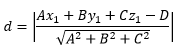This equation gives us the perpendicular distance of a point from a plane, using the Cartesian Method.

To learn how to calculate the shortest distance or the perpendicular distance of a point from a plane using the Vector Method and the Cartesian Method, download Byju’s- The Learning App.